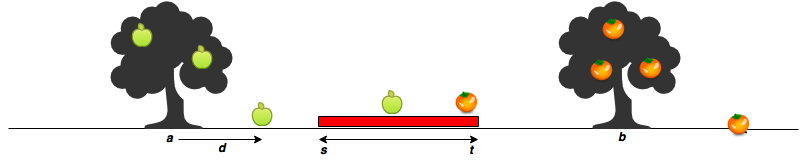Sam's house has an apple tree and an orange tree that yield an abundance of fruit. Using the information given below, determine the number of apples and oranges that land on Sam's house.

In the diagram below:

• The red region denotes the house, where  is the start point, and  is the endpoint. The apple tree is to the left of the house, and the orange tree is to its right.
• Assume the trees are located on a single point, where the apple tree is at point , and the orange tree is at point .
• When a fruit falls from its tree, it lands  units of distance from its tree of origin along the -axis. *A negative value of  means the fruit fell  units to the tree's left, and a positive value of  means it falls  units to the tree's right. *Given the value of  for  apples and  oranges, determine how many apples and oranges will fall on Sam's house (i.e., in the inclusive range )?

For example, Sam's house is between  and . The apple tree is located at  and the orange at . There are  apples and  oranges. Apples are thrown  units distance from , and  units distance. Adding each apple distance to the position of the tree, they land at . Oranges land at . One apple and two oranges land in the inclusive range  so we print

1
2


Function Description

Complete the countApplesAndOranges function in the editor below. It should print the number of apples and oranges that land on Sam's house, each on a separate line.

countApplesAndOranges has the following parameter(s):

• s: integer, starting point of Sam's house location.
• t: integer, ending location of Sam's house location.
• a: integer, location of the Apple tree.
• b: integer, location of the Orange tree.
• apples: integer array, distances at which each apple falls from the tree.
• oranges: integer array, distances at which each orange falls from the tree.

Input Format

The first line contains two space-separated integers denoting the respective values of  and .
The second line contains two space-separated integers denoting the respective values of  and .
The third line contains two space-separated integers denoting the respective values of  and .
The fourth line contains  space-separated integers denoting the respective distances that each apple falls from point .
The fifth line contains  space-separated integers denoting the respective distances that each orange falls from point .

Constraints

Output Format

Print two integers on two different lines:

1. The first integer: the number of apples that fall on Sam's house.
2. The second integer: the number of oranges that fall on Sam's house.

Sample Input 0

7 11
5 15
3 2
-2 2 1
5 -6


Sample Output 0

1
1


Explanation 0

The first apple falls at position .
The second apple falls at position .
The third apple falls at position .
The first orange falls at position .
The second orange falls at position .
Only one fruit (the second apple) falls within the region between  and , so we print  as our first line of output.
Only the second orange falls within the region between  and , so we print  as our second line of output.

c++ implementation:

void countApplesAndOranges(int s, int t, int a, int b, vector<int>ap, vector<int> og)
{
int m= ap.size();
int n=og.size();
int c1=0; int c2=0;
for(int i=0;i<m;i++)
{
if(ap[i]+a>=s && ap[i]+a<=t)
c1++;
}
for(int i=0;i<n;i++)
{
if( og[i]+b>=s && og[i]+b<=t)
c2++;
}
cout<<c1<<endl;
cout<<c2<<endl;
}

Time Complexity: O(n)
space Complexity: O(1)

darkmode